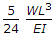# Civil Engineering - Strength of Materials - Discussion

### Discussion :: Strength of Materials - Section 3 (Q.No.2)

2.

A cantilever carries is uniformly distributed load W over its whole length and a force W acts at its free end upward. The net deflection of the free end will be

 [A]. zero [B].upward [C].downward [D]. none of these.

Explanation:

No answer description available for this question.

 Lee said: (Jun 4, 2016) Net deflection= WL^3/8EI - WL^3/3EI. = 5WL^3/24EI.

 Rishi said: (Jul 13, 2017) Deflection of Cantilever in UDL is wl^4/8EI. HOW COME WL^3/8EI?

 Sabibur Rahaman said: (Jul 21, 2017) Support always deflection zero. This type of beam propped cantilever. And cantilever beam UDL load come deflection wl^4/8EI or Points load come deflection wl^3/3EI.

 Dhanu said: (Sep 13, 2017) Net deflection= WL^3/3EI - (wl)L^3/8EI. 5/24 WL^3/EI.

 Ram Vamne said: (Sep 18, 2017) Deflection always down word? How to upward?

 Ankit said: (Dec 22, 2017) There is a reaction at the free end i.e. (3wl)/8 it causes upward deflection @Ram.

 Pratik said: (Aug 29, 2018) @Rishi. W= w*l.

 Muhammad Zeeshan Akram said: (Dec 11, 2018) UDL=1/8 & Concentrated Load=1/8. Take difference of both because they are in opposite direction we will get the required Answer B.

 Sagar Rathor said: (Jan 8, 2019) In this Question, given UDL is already converted in point load W. So we have to take WL^3/8EI in place of wL^4/8EI.

 Ashish Sharma said: (Sep 19, 2020) Where CONSIDER. wl^4/8EI = + (because downward). wl^3/3EI= - (BECAUSE UPWARD). Then; WL^4/8EI -WL^3/3EI. WHERE CONSIDER. W=L=1 ,, EI =IS CONSTANT FOR BOTH. Put the value. ANSWER IS NEGATIVE (-5/24). I consider minus sign is upward direction. SO ANSWER IS UPWARD.